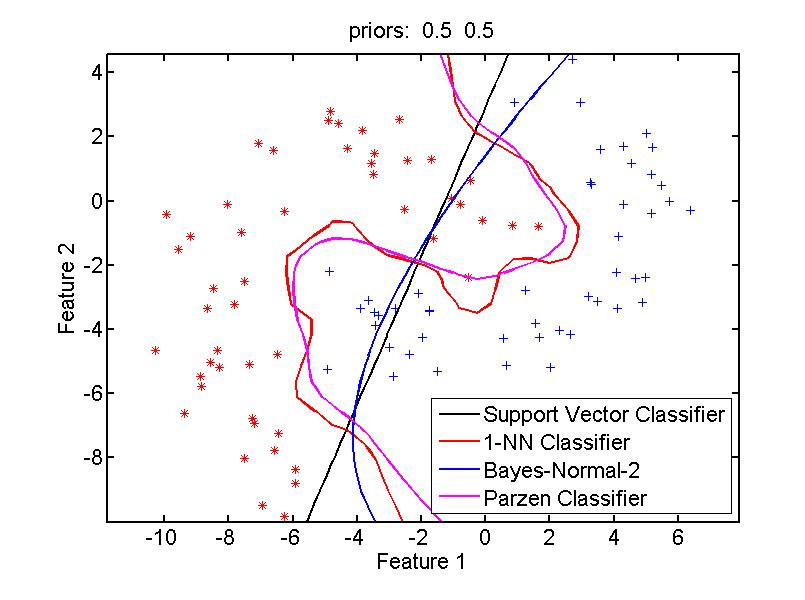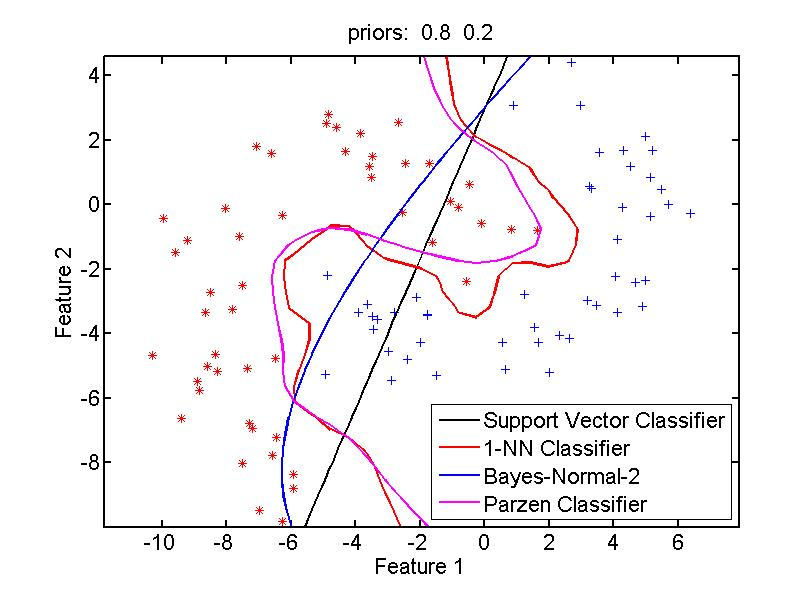### Why and when to set prior probabilities in dataset?

By default the prior probabilities in dataset are unset (empty). When needed PRTools takes the class frequencies in the dataset as priors. If it is known that the class priors are different they should be set. Not all classifiers and evaluation routines are sensitive for that. E.g. the support vector classifier (svc) and the nearest neighbor rule (knnc) are not as they focus on the distances in  the data. Density based classifiers on the contrary are sensitive to the priors are they directly change posterior probabilities. See the below example.

`>> w1 = svc;`
`>> w2 = knnc([],1);`
`>> w3 = qdc;`
`>> w4 = parzendc;`
`>> a = gendatb;`
`>> a = setprior(a,[0.5 0.5]);`
`>> figure(1); scatterd(a);`
`>> plotc(a*{w1,w2,w3,w4})`
`>> title(['priors:  '  num2str(getprior(a),'%5.1f')])`
`>> b = setprior(a,[0.8 0.2]);`
`>> figure(2); scatterd(b);`
`>> plotc(b*{w1,w2,w3,w4})`
`>> title(['priors:  '  num2str(getprior(b),'%5.1f')])`

Note that the boundaries for the support vector classifier and the nearest neighbor rule are identical for the two figures. The density based classifiers assuming normal distributions and using the Parzen estimator shift into the direction to the red `*` class (lower prior) and away from the blue `+` class (higher prior).